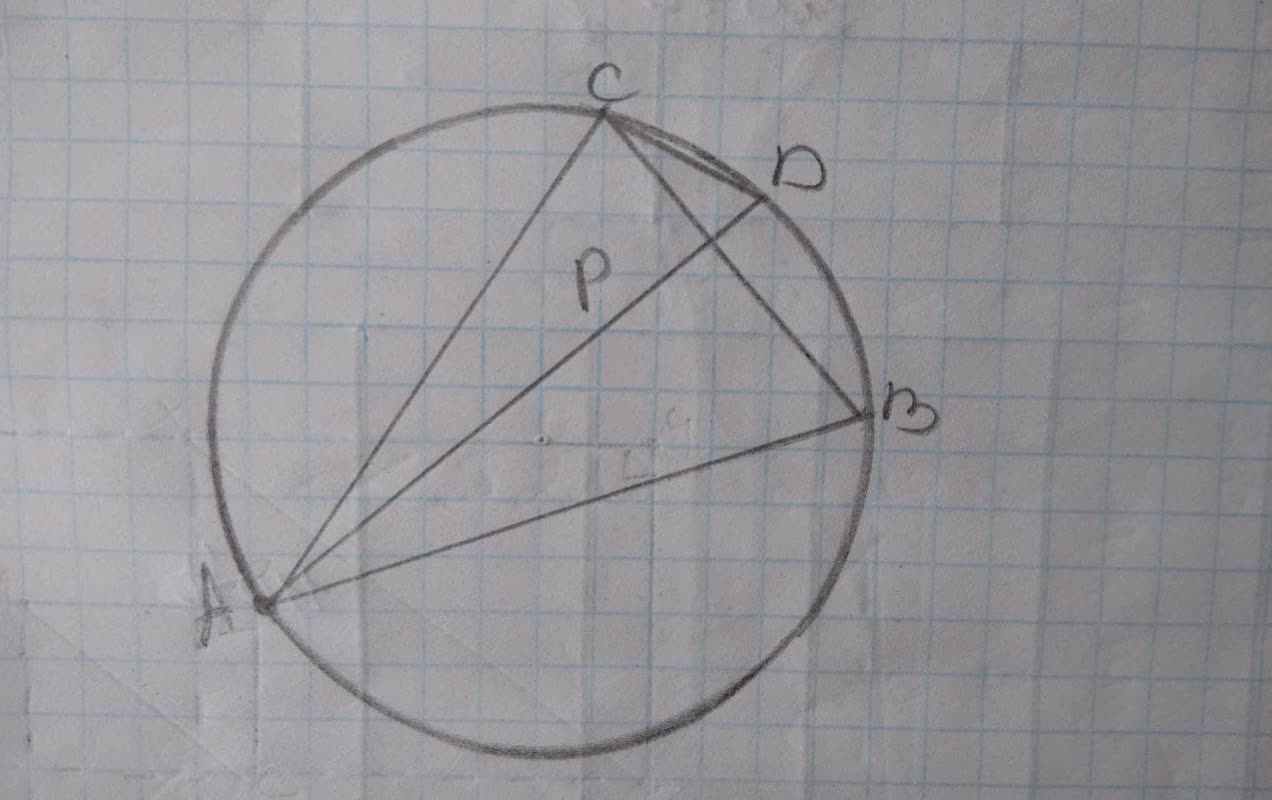## ABC is an inscribed triangle and chord AD bisects angle A and cuts BC at P. Prove: (AD)/(CD)=(AC)/(PC).

TrigonometryABC is an inscribed triangle and chord AD bisects angle A and cuts BC at P. Prove: (AD)/(CD)=(AC)/(PC).2 years ago
ABC is an inscribed triangle and chord AD bisects angle A and cuts BC at P. Prove: (AD)/(CD)=(AC)/(PC).
GIven: Inscribed △ ABC, chord AD bisects ∠ A and cuts BC at P; chord CD
Statements
1.(see above)
2. ∠ D=∠ D
3.
4. ∠ BCD=∠ DAB
5.
6. △ ACD-△ CPD
Step1 ∠D=∠ D Here angle D is equal to itself, that is reflexive property. Given, AD bisects angle A, so by definition of angle bisector ∠ CAD=∠ DAB Step2 From the picture, we see that angle BCD and angle DAB are two inscribed angles with the same base points on the circle. So they are equal. ∠ BCD=∠DAB If we substitute angle DAB by angle BCD in ∠ CAD=∠ DAB We get: ∠ BCD=∠ CAD So triangle ACD and triangle CPD has two pairs of angles equal. So they are ~ilar by AA property.Corollary 57-1 If two angles of one triangle are equal respectively to two angles of another, then the triangles are ~ilar. (a.a.) So, (AD)/(CD)=(AC)/(PC) by C.S.S.T.P, - corresponding sides of ~ilar triangles are proportional. Answer: 2) Reflexive property 3) ∠ CAD=∠ DAB 4)Inscribed angles with same base points 5) ∠ BCD=∠ CAD 6) a.a 7) C.S.S.T.P.